# Machine Learning - Supervised

#### Machine Learning & BIG Data Analytics: Microsoft AZURE

46 Lectures 3.5 hours

#### Practical Machine Learning using Python

Best Seller

91 Lectures 23.5 hours

#### Machine Learning with Python (beginner to guru)

Most Popular

30 Lectures 24 hours

Supervised learning is one of the important models of learning involved in training machines. This chapter talks in detail about the same.

## Algorithms for Supervised Learning

There are several algorithms available for supervised learning. Some of the widely used algorithms of supervised learning are as shown below −

• k-Nearest Neighbours
• Decision Trees
• Naive Bayes
• Logistic Regression
• Support Vector Machines

As we move ahead in this chapter, let us discuss in detail about each of the algorithms.

## k-Nearest Neighbours

The k-Nearest Neighbours, which is simply called kNN is a statistical technique that can be used for solving for classification and regression problems. Let us discuss the case of classifying an unknown object using kNN. Consider the distribution of objects as shown in the image given below −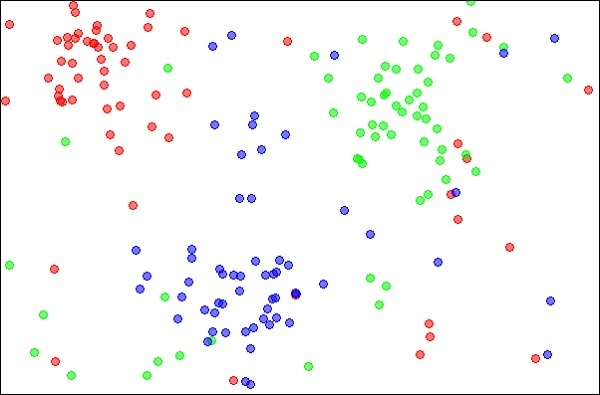Source:

https://en.wikipedia.org/wiki/K-nearest_neighbors_algorithm

The diagram shows three types of objects, marked in red, blue and green colors. When you run the kNN classifier on the above dataset, the boundaries for each type of object will be marked as shown below −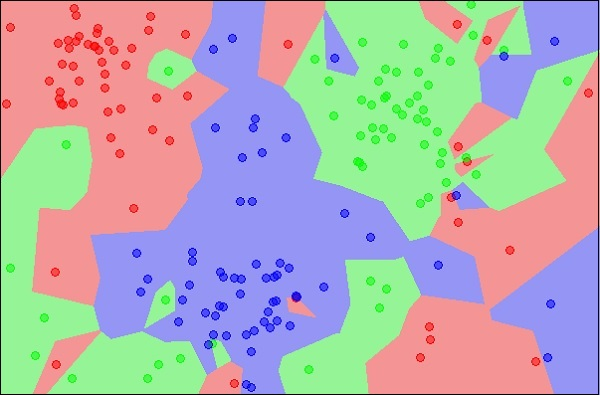Source:

https://en.wikipedia.org/wiki/K-nearest_neighbors_algorithm

Now, consider a new unknown object that you want to classify as red, green or blue. This is depicted in the figure below.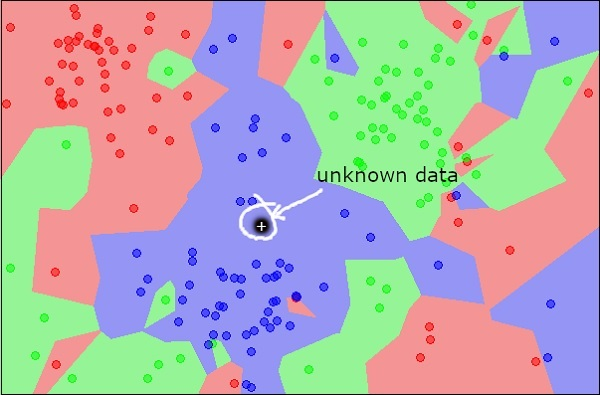As you see it visually, the unknown data point belongs to a class of blue objects. Mathematically, this can be concluded by measuring the distance of this unknown point with every other point in the data set. When you do so, you will know that most of its neighbours are of blue color. The average distance to red and green objects would be definitely more than the average distance to blue objects. Thus, this unknown object can be classified as belonging to blue class.

The kNN algorithm can also be used for regression problems. The kNN algorithm is available as ready-to-use in most of the ML libraries.

## Decision Trees

A simple decision tree in a flowchart format is shown below −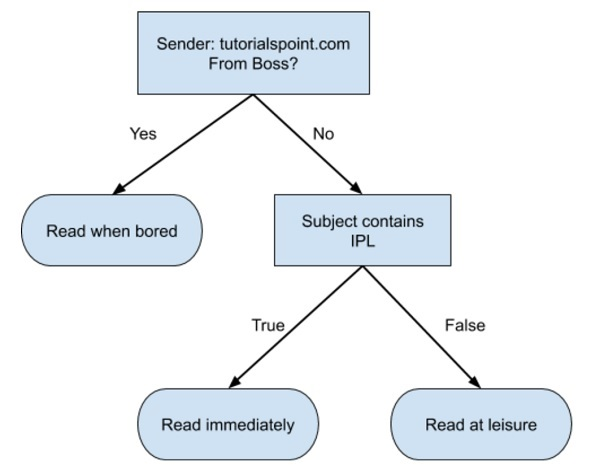You would write a code to classify your input data based on this flowchart. The flowchart is self-explanatory and trivial. In this scenario, you are trying to classify an incoming email to decide when to read it.

In reality, the decision trees can be large and complex. There are several algorithms available to create and traverse these trees. As a Machine Learning enthusiast, you need to understand and master these techniques of creating and traversing decision trees.

## Naive Bayes

Naive Bayes is used for creating classifiers. Suppose you want to sort out (classify) fruits of different kinds from a fruit basket. You may use features such as color, size and shape of a fruit, For example, any fruit that is red in color, is round in shape and is about 10 cm in diameter may be considered as Apple. So to train the model, you would use these features and test the probability that a given feature matches the desired constraints. The probabilities of different features are then combined to arrive at a probability that a given fruit is an Apple. Naive Bayes generally requires a small number of training data for classification.

## Logistic Regression

Look at the following diagram. It shows the distribution of data points in XY plane.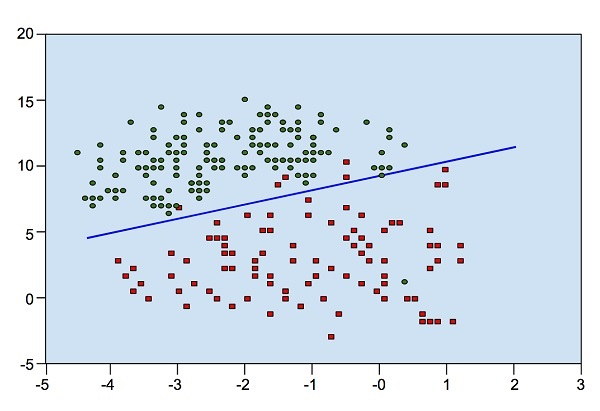From the diagram, we can visually inspect the separation of red dots from green dots. You may draw a boundary line to separate out these dots. Now, to classify a new data point, you will just need to determine on which side of the line the point lies.

## Support Vector Machines

Look at the following distribution of data. Here the three classes of data cannot be linearly separated. The boundary curves are non-linear. In such a case, finding the equation of the curve becomes a complex job.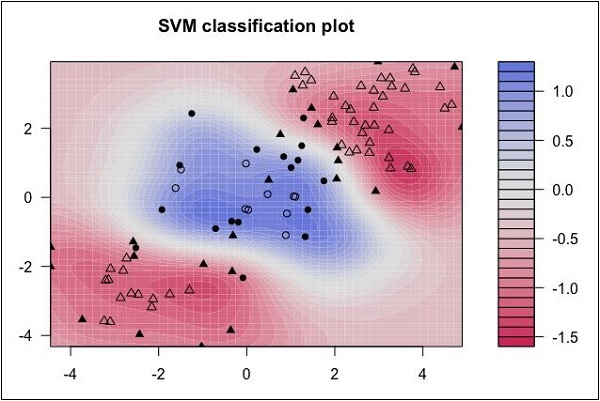Source: http://uc-r.github.io/svm

The Support Vector Machines (SVM) comes handy in determining the separation boundaries in such situations.